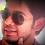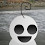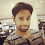### Lets think Mathematics!

Lets get geeky!
Did you know? - My mom is a maths teacher! Well, it doesn't mean anything like I'm a genius in maths :P . In this vacation the Kerala government is conducting funny courses to the teachers in all subjects to train them the new aspects of revised syllabus. The teachers are actually divided into groups, given assignments, some tricky questions and even homeworks!

Now today.. mom came up with two tricky questions.

Q #1
Take 4 numbers.
The sum of the first two numbers should be double the sum of the other two.
But the product of the first two should be half the product of the other two.

Actually we can find more than one set of such numbers.
Eg: 33 1, 11 6
33+1=34
11+6=17=2 x 34

33 x 1 = 33
11 x 6 = 66 =2 x 33

Got it?!

To solution..
Okey, so we burned our brains on various combinations of numbers, looking out for general properties and all. Not to mention we ended up with nothing more. :(

And then this thought struck me "Why don't I leave it to the computer?"
I wrote a very simple program in Java and amazingly the computer showed me a list of such numbers satisfying the above condition!

Below is the algorithm behind it..

for(a=1;a<=50;a++)
for(b=1;b<=100;b++)
for(p=1;p<=50;p++)
for(q=1;q<=2*p;q++)
if(a+b==2.0*(p+q) && a*b==p*q/2.0)
// Print a,b, c,d

The numbers that we needed(below 100) are..
1 33 , 6 11
1 45 , 18 5
2 60 , 15 16
2 66 , 12 22
2 90 , 36 10
3 91 , 21 26
3 95 , 30 19
3 99 , 33 18

When was I ever gonna find 'em without my computer? After all.. studying Java at school proves a little useful! :O

Q #2
Let us think (a + b) x a x b = 4545
The question is that "Are a and b natural numbers?"

To solution..
This time I left it to the computer with no other option!

The idea was to check all combinations of a and b where I ranged a from 1 to 50, and b from 1 to 200. But none of them gave 4545. So they must be some fractions!! :D

Now I made a program to check fractions in an attempt to find a and b. But I failed in this attempt. So far no such a and b exists :( .

Can you find them??

The algorithm used to find a and b is as follows..

for(a=.01;a<=50;a+=0.001)
for(b=1;b<=200;b+=0.001)
if((a+b)*a*b==4545)
//Print a,b

//Print "Over!"

I really think no such numbers exist!

1.Ugh!!! I don't deserve this kinda punishment for taking a break! Sheeesh..
Didn't read through it at all :P
Have had enough with any kind of activity that involves usage of the brain.....
& ur mum's a math teach???? (shiver) :~ :f

2.Oh my GOD! Yes, I also do not expect to read maths during my free time!

I also skipped reading your maths parts Sir Et. Sorry, but Maths and me are not best friends.

3.This ( Q#2 ) is quite a simple problem.
you want solutions for (a+b) ab = 4545.
Since you need a solution from set of natural numbers both a and b should be natural numbers , so is (a+b) and (ab). So this is equivalent to asking can you find two numbers whose product is 4545 ( With an extra restriction though ). So the possible solutions are obtained by exhaustively listing factors of 4545. Which are {1,4545}, {3,1515} , {9,55}. Now you can check easily that none of these pairs can be sum and product of two natural numbers. Hence the proof. To the second part of the question , a solution from Real Domain is very simple. Lets say a=b=c. Then we have to find the solution of ( c + c ) * ( c c ) = 4545 which is 2 c * ( c^2 ) = 4545 which gives c = CubeRoot(4545/2).

4.Whoa! This is a typical geek post!

P.S. I have always wanted to use this smiley! It's so adorable! :e

5.:@ :v :e no maths et !

6.@ Sajin
Wowy that makes a nice proof! The answer comes around 13.something which actually gives 4544.999... well chalega, anyhow!

@ all
Aww aww.. i'm so sorry. The post was a quicky one and didn't really think about all you people looking for something relaxing in your free times! Totally stopped mathematics here!!

@ choco
Study well oye.. stop peeping into blogs!

7.Errr....pardon my ignorance!!....but one of the main reasons I didn't take up engineering is due to maths.I might even break out into an allergic reaction if I come anywhere near it..hence I skipped the maths part!!
But I'm sure....err....that's its an interesting problem!!!...Rather..."gasp"...fascinating!!!... :P

8.:b
U an ICSE student?
even though i have studied Java recently in my 10th, I would have never thought to look into Java!
You are so very geeky!

9.@ Vyazz
LOLZ buddy! maths-alergy.. ah?! :O

@ ramya
I'm not an ICSE student!! Why, only ICSE students can handle maths?? :d
Oh my.. Java in 10th. Sorry 'm not at all geeky to take up Java in 10th!! :D

10.LOL..a post on math... well thats brave of u... i dn't read the whole thing though..exams going on..dnt need more problems to tackle with.. :P

11.@ Nim
yeah yeah.. i can understand! :O